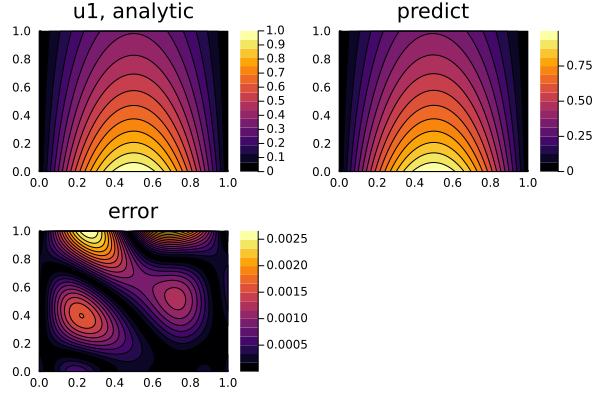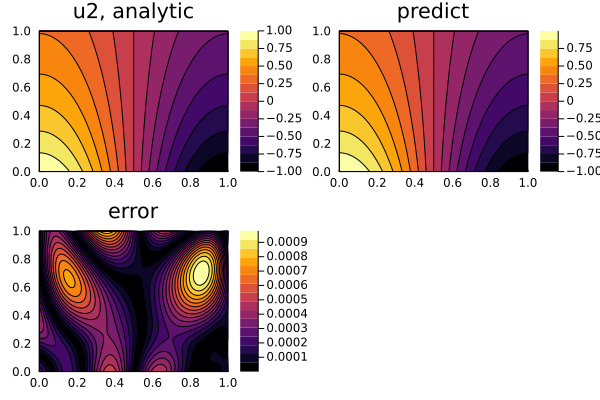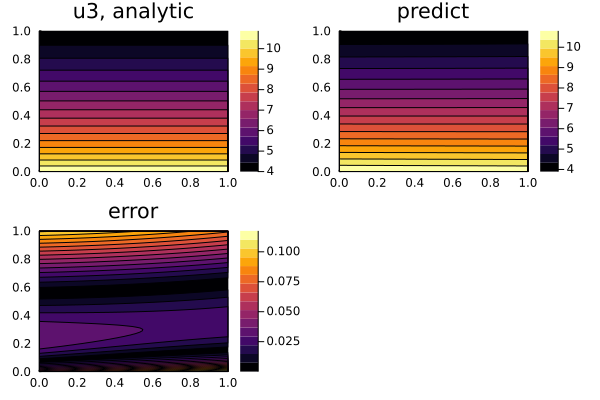# Defining Systems of PDEs for Physics-Informed Neural Networks (PINNs)

In this example, we will solve the PDE system:

\begin{align*} ∂_t u_1(t, x) & = ∂_x^2 u_1(t, x) + u_3(t, x) \, \sin(\pi x) \, ,\\ ∂_t u_2(t, x) & = ∂_x^2 u_2(t, x) + u_3(t, x) \, \cos(\pi x) \, ,\\ 0 & = u_1(t, x) \sin(\pi x) + u_2(t, x) \cos(\pi x) - e^{-t} \, , \end{align*}

with the initial conditions:

\begin{align*} u_1(0, x) & = \sin(\pi x) \, ,\\ ∂_t u_1(0, x) & = - \sin(\pi x) \, ,\\ u_2(0, x) & = \cos(\pi x) \, ,\\ ∂_t u_2(0, x) & = - \cos(\pi x) \, , \end{align*}

and the boundary conditions:

\begin{align*} u_1(t, 0) & = u_1(t, 1) = 0 \, ,\\ u_2(t, 0) & = - u_2(t, 1) = e^{-t} \, , \end{align*}

with physics-informed neural networks.

## Solution

using NeuralPDE, Lux, ModelingToolkit, Optimization, OptimizationOptimJL
import ModelingToolkit: Interval, infimum, supremum

@parameters t, x
@variables u1(..), u2(..), u3(..)
Dt = Differential(t)
Dtt = Differential(t)^2
Dx = Differential(x)
Dxx = Differential(x)^2

eqs = [Dtt(u1(t,x)) ~ Dxx(u1(t,x)) + u3(t,x)*sin(pi*x),
Dtt(u2(t,x)) ~ Dxx(u2(t,x)) + u3(t,x)*cos(pi*x),
0. ~ u1(t,x)*sin(pi*x) + u2(t,x)*cos(pi*x) - exp(-t)]

bcs = [u1(0,x) ~ sin(pi*x),
u2(0,x) ~ cos(pi*x),
Dt(u1(0,x)) ~ -sin(pi*x),
Dt(u2(0,x)) ~ -cos(pi*x),
u1(t,0) ~ 0.,
u2(t,0) ~ exp(-t),
u1(t,1) ~ 0.,
u2(t,1) ~ -exp(-t)]

# Space and time domains
domains = [t ∈ Interval(0.0,1.0),
x ∈ Interval(0.0,1.0)]

# Neural network
input_ = length(domains)
n = 15
chain =[Lux.Chain(Dense(input_,n,Lux.σ),Dense(n,n,Lux.σ),Dense(n,1)) for _ in 1:3]

discretization = PhysicsInformedNN(chain, strategy)

@named pdesystem = PDESystem(eqs,bcs,domains,[t,x],[u1(t, x),u2(t, x),u3(t, x)])
prob = discretize(pdesystem,discretization)
sym_prob = symbolic_discretize(pdesystem,discretization)

pde_inner_loss_functions = sym_prob.loss_functions.pde_loss_functions
bcs_inner_loss_functions = sym_prob.loss_functions.bc_loss_functions

callback = function (p, l)
println("loss: ", l)
println("pde_losses: ", map(l_ -> l_(p), pde_inner_loss_functions))
println("bcs_losses: ", map(l_ -> l_(p), bcs_inner_loss_functions))
return false
end

res = Optimization.solve(prob,BFGS(); callback = callback, maxiters=5000)

phi = discretization.phi

## Direct Construction via symbolic_discretize

One can take apart the pieces and reassemble the loss functions using the symbolic_discretize interface. Here is an example using the components from symbolic_discretize to fully reproduce the discretize optimization:

using NeuralPDE, Lux, ModelingToolkit, Optimization, OptimizationOptimJL
import ModelingToolkit: Interval, infimum, supremum

@parameters t, x
@variables u1(..), u2(..), u3(..)
Dt = Differential(t)
Dtt = Differential(t)^2
Dx = Differential(x)
Dxx = Differential(x)^2

eqs = [Dtt(u1(t,x)) ~ Dxx(u1(t,x)) + u3(t,x)*sin(pi*x),
Dtt(u2(t,x)) ~ Dxx(u2(t,x)) + u3(t,x)*cos(pi*x),
0. ~ u1(t,x)*sin(pi*x) + u2(t,x)*cos(pi*x) - exp(-t)]

bcs = [u1(0,x) ~ sin(pi*x),
u2(0,x) ~ cos(pi*x),
Dt(u1(0,x)) ~ -sin(pi*x),
Dt(u2(0,x)) ~ -cos(pi*x),
u1(t,0) ~ 0.,
u2(t,0) ~ exp(-t),
u1(t,1) ~ 0.,
u2(t,1) ~ -exp(-t)]

# Space and time domains
domains = [t ∈ Interval(0.0,1.0),
x ∈ Interval(0.0,1.0)]

# Neural network
input_ = length(domains)
n = 15
chain =[Lux.Chain(Dense(input_,n,Lux.σ),Dense(n,n,Lux.σ),Dense(n,1)) for _ in 1:3]
@named pdesystem = PDESystem(eqs,bcs,domains,[t,x],[u1(t, x),u2(t, x),u3(t, x)])

discretization = PhysicsInformedNN(chain, strategy)
sym_prob = NeuralPDE.symbolic_discretize(pdesystem, discretization)

pde_loss_functions = sym_prob.loss_functions.pde_loss_functions
bc_loss_functions = sym_prob.loss_functions.bc_loss_functions

callback = function (p, l)
println("loss: ", l)
println("pde_losses: ", map(l_ -> l_(p), pde_loss_functions))
println("bcs_losses: ", map(l_ -> l_(p), bc_loss_functions))
return false
end

loss_functions =  [pde_loss_functions;bc_loss_functions]

function loss_function(θ,p)
sum(map(l->l(θ) ,loss_functions))
end

f_ = OptimizationFunction(loss_function, Optimization.AutoZygote())
prob = Optimization.OptimizationProblem(f_, sym_prob.flat_init_params)

res = Optimization.solve(prob,OptimizationOptimJL.BFGS(); callback = callback, maxiters=5000)

## Solution Representation

Now let's perform some analysis for both the symbolic_discretize and discretize APIs:

using Plots

phi = discretization.phi
ts,xs = [infimum(d.domain):0.01:supremum(d.domain) for d in domains]

minimizers_ = [res.u.depvar[sym_prob.depvars[i]] for i in 1:3]

analytic_sol_func(t,x) = [exp(-t)*sin(pi*x), exp(-t)*cos(pi*x), (1+pi^2)*exp(-t)]
u_real  = [[analytic_sol_func(t,x)[i] for t in ts for x in xs] for i in 1:3]
u_predict  = [[phi[i]([t,x],minimizers_[i]) for t in ts  for x in xs] for i in 1:3]
diff_u = [abs.(u_real[i] .- u_predict[i] ) for i in 1:3]
for i in 1:3
p1 = plot(ts, xs, u_real[i],linetype=:contourf,title = "u$i, analytic"); p2 = plot(ts, xs, u_predict[i],linetype=:contourf,title = "predict"); p3 = plot(ts, xs, diff_u[i],linetype=:contourf,title = "error"); plot(p1,p2,p3) savefig("sol_u$i")
endNotice here that the solution is represented in the OptimizationSolution with u as the parameters for the trained neural network. But, for the case where the neural network is from Lux.jl, it's given as a ComponentArray where res.u.depvar.x corresponds to the result for the neural network corresponding to the dependent variable x, i.e. res.u.depvar.u1 are the trained parameters for phi in our example. For simpler indexing, you can use res.u.depvar[:u1] or res.u.depvar[Symbol(:u,1)] as shown here.

Subsetting the array also works, but is inelegant.

(If param_estim == true, then res.u.p are the fit parameters)

If Flux.jl is used, then subsetting the array is required. This looks like:

init_params = [Flux.destructure(c) for c in chain]
acum =  [0;accumulate(+, length.(init_params))]
sep = [acum[i]+1 : acum[i+1] for i in 1:length(acum)-1]
minimizers_ = [res.minimizer[s] for s in sep]

#### Note: Solving Matrices of PDEs

Also, in addition to vector systems, we can use the matrix form of PDEs:

using ModelingToolkit, NeuralPDE
@parameters x y
@variables u[1:2,1:2](..)
@derivatives Dxx''~x
@derivatives Dyy''~y

# Initial and boundary conditions
bcs = [u(x,0) ~ x, u(x,0) ~ 2, u(x,0) ~ 3, u(x,0) ~ 4]

# matrix PDE
eqs  = @. [(Dxx(u_(x,y)) + Dyy(u_(x,y))) for u_ in u] ~ -sin(pi*x)*sin(pi*y)*[0 1; 0 1]

size(eqs)
(2, 2)